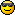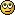#### Topic: How to compute astrophysical reaction rates from experimental data

Usually, reaction cross sections or astrophysical S-factors are quoted in experimental papers. In order to use the data in astrophysical calculations, it is necessary to convert the given values to astrophysical reaction rates. This is not straightforward because there are two problematic points:

1) The data usually cover only a limited energy range and may be largely spaced;
2) error bars have to be considered.

These points pose difficulties for computing the rate by numerically solving the relevant integral (see, e.g., the definition of the nucleus-nucleus reaction rate).

In order to facilitate the task I provide the FORTRAN 90/95 program 'exp2rate.f90' which automatically takes care of these points.

The main features of the program are:

1) Cross sections or S-factors can be used as input. If cross sections are given, the S-factors are additionally computed and written to file. Internally, S-factors are used in the integration for better accuracy.

2) The data is interpolated in the integration. It can be chosen whether a linear or a spline interpolation is used.

3) The rates are only computed for a valid range of temperatures defined by the data. The valid temperature range is automatically determined from numerical considerations.4) The experimental error bars on both data and energy are taken into account. This yields a rate with error bars!Experimentalists are especially invited to make use of this program to provide derived reaction rates with error bars in their papers.

For further details see the instructions contained in the comment section at the beginning of the program.

Special note: Sometimes the approximate formula for the Gamow peak given in Eqs. 4.21 and 4.25 of the Rolfs & Rodney book "Cauldrons in the Cosmos" is used to determine the relevant energy/temperature range. However, this is an approximation which decreases in accuracy with increasing charge of the particles and only applies for constant S-factors! The above program numerically determines the actual Gamow peak using the provided data and therefore is more accurate.#### Re: How to compute astrophysical reaction rates from experimental data

New version available featuring improved accuracy handling.

The format of the input file 'rate.in' has slightly changed. Please adapt it to the new version.
In the input file, the desired accuracy of the rate can be chosen. It can also be chosen whether the computed rate should be adjusted by the estimate for the accuracy.

Technical detail: The accuracy is determined by extrapolating data outside the given energy range and checking whether this has any impact on the integration. It is just an estimate because it is based on an extrapolation.

#### Re: How to compute astrophysical reaction rates from experimental data

Physical Review C 75, 045801 (2007)

This interesting paper points out that the usual Gamow peak concept cannot be applied to resonant reactions (considering examples of charged particle capture on light nuclei). It appears that strong, narrow resonances from even below the Gamow peak may contribute.

This further underlines the importance for using 'exp2rate.f90' which determines the relevant energy region from purely numerical considerations.
(It should be noted, however, that values outside of the given data range are extrapolated assuming that there are no further resonances.)

Additional note: (see also the special note in the How To above) The usual Gamow peak approximation given in textbooks and repeated in the above paper is also increasingly inaccurate for increasingly charged target nuclei, even in the non-resonant case. For example, this can be shown in a comparison of the approximation and the integrals obtained in Hauser-Feshbach calculations. The location of the maximum contribution (the Gamow "peak") is increasingly shifted with respect to the approximation. Also the shape becomes less and less Gaussian.

#### Re: How to compute astrophysical reaction rates from experimental data

Physical Review C 81, 045807 (2010)

Finally, I have found time (and deemed it sufficiently important because a lot of experiments were designed around the insufficient Gamow peak approximation) to produce a large-scale study of the astrophysically relevant energy windows. The above paper shows that for a large number of nuclei the relevant energy windows are shifted by several MeV with respect to the ones predicted by the standard formula.

The paper also provides an extended table of improved energy windows to be used for the determination of astrophysically important energy ranges.

I hope this is interesting and useful for experimentalists and theorists alike.

The code 'exp2rate.f90' determines the relevant energy region from purely numerical considerations and therefore automatically includes the correct treatment of the Gamow window (using the actual data). Therefore, the above table does not have to be consulted additionally when using this code.